# How to Calculate MACD in Excel

Learn how to calculate and plot MACD in Excel, and start making better trading decisions.

The Moving Average Convergence Divergence (or MACD) indicator is a powerful momentum-based trading indicator.

This article is the first of a two-part series. This part offers a step-by-step guide to calculating and charting MACD in Excel. The second part explores how market technicians use MACD to make better trading decisions.

An MACD chart consists of three elements. The first is the difference between the 12-day and 26-day exponential moving average (EMA) of the closing price; this is the MACD line. The second is the  EMA of the difference; this is the signal line. The third is simply the MCAD minus the signal, and is known as the histogram.

The chart below, for example, is the MACD and signal line for Apple between two dates.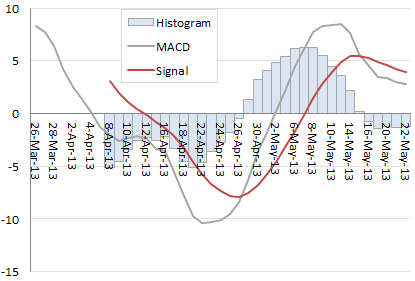A buy signal is generated when a rising MACD crosses over the the signal line (i.e. when the histogram goes from negative to positive). A sell signal, however, is generated when a falling MACD crosses over the signal line (i.e. when the histogram goes from positive negative). Other nuances will be explored in the next article in this series.

Developed by Gerald Appel in the 1970s, MACD is now widely used by traders to generate forecast price trends, and generate buy and sell signals.

In the following step-by-step guide, we’ll calculate the MACD of Apple, giving you all the tools you need to recreate the chart above. You can download the complete spreadsheet at the bottom of this article.

Step 1: Get historical daily close prices

For the worked example below, we use daily close prices for Apple (ticker: AAPL) from 19th Feb 2013 to 22nd May 2013; dates are in Column A and prices in Column C.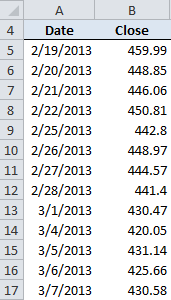Step 2. 12-day EMA of the close prices

The first value is simply a trailing 12-day average, calculated with Excel’s =AVERAGE() function. All other values are given by this formula.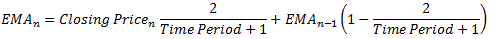where Time Period is 12, n refers to today, and n-1 refers to yesterday. Essentially, today’s EMA is a function of today’s closing price and yesterday’s EMA.

The screengrab below illustrates what the spreadsheet should look like, and how the formulas are entered.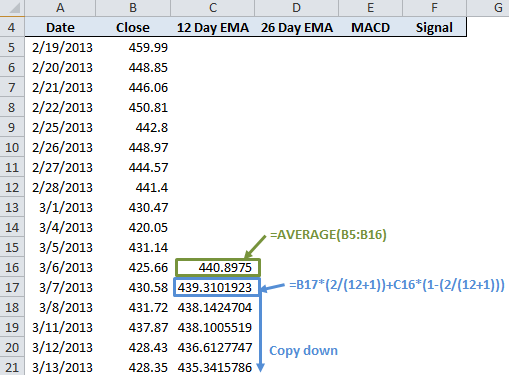Step 3. 26-day EMA of the close prices

Again, the first value is simply an average of the last 26 day’s closing prices, with all other values given by the above formula (with the Time Period equal to 26)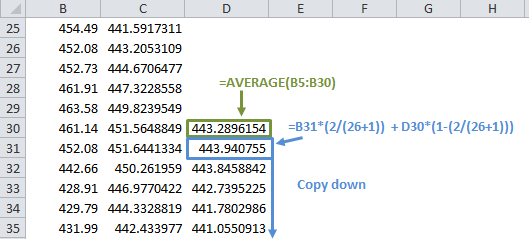Step 4. Calculate the MACD

The MACD is simply the 12 day EMA minus the 26 day EMA.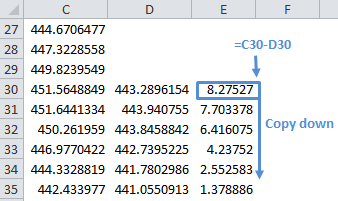Step 5. The signal line

This is a 9-day EMA of the MACD. The first value is simply a 9-day trailing average. All other values are given by this equation, where the time period is 9.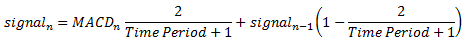This is what the signal calculation should look like in Excel.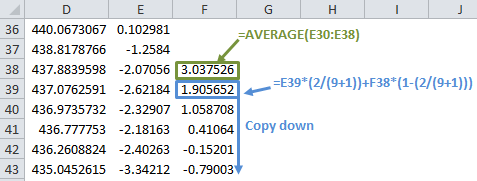You now have all the data you need! With Excel’s charting tools, you can now plot the 12- and 26-day EMA, MACD and signal data.

You can cross-check the results against those produced by Yahoo Finance; both this spreadsheet and Yahoo Finance should give the same MACD.### 9 thoughts on “How to Calculate MACD in Excel”

1. Excellent looking spreadsheet. I also referenced your other work to get real time price data (My application is forex) constantly updated. My question is, Now how do you combine these two ideas so that when a new price point is added/ updated, it automatically calculates the newest MACD (and associated EMA’s)?

• Hi Samir, many thanks for this excellent spreadsheet. I would like to second John’s request – albeit for equity prices and not forex.

2. Please tell me how to use the chart function in Excel to make my chart look like the one in this article.

• Experiment and explore. It’s not difficult if you’re motivated.

3. Hi Samir,

I’ve tried using your tutorial and the bulk downloading spreadsheet. When I open your tutorial the numbers all look like they are supposed to but when I type in the formula or even cut and paste the numbers all become identical. I looked at the calculations in the cells and they are the same as you had in the tutorial sheet. What could I be doing wrong?